# Solution Properties Solution Composition The Energies of Solution

• Slides: 76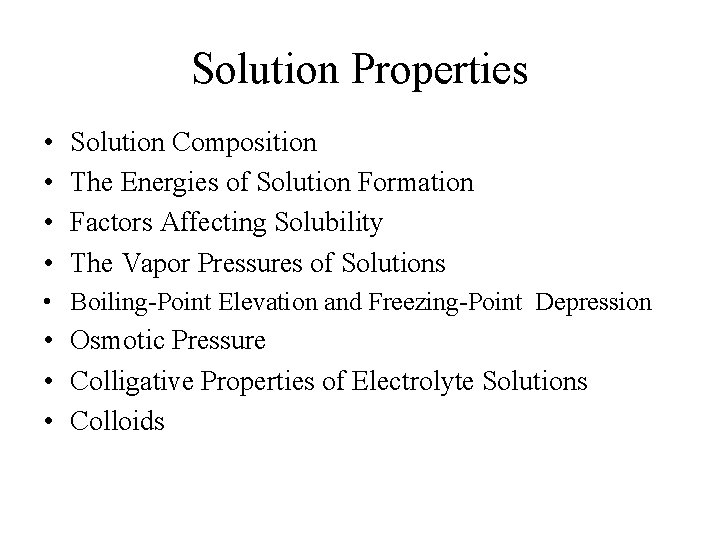Solution Properties • • Solution Composition The Energies of Solution Formation Factors Affecting Solubility The Vapor Pressures of Solutions • Boiling-Point Elevation and Freezing-Point Depression • Osmotic Pressure • Colligative Properties of Electrolyte Solutions • ColloidsVarious Types of Solutions Example State of Solution State of Solute State of Solvent Air, natural gas Gas Gas Vodka, antifreeze Liquid Brass Solid Carbonated water (soda) Liquid Gas Liquid Seawater, sugar solution Liquid Solid Liquid Hydrogen in platinum Solid Gas Solid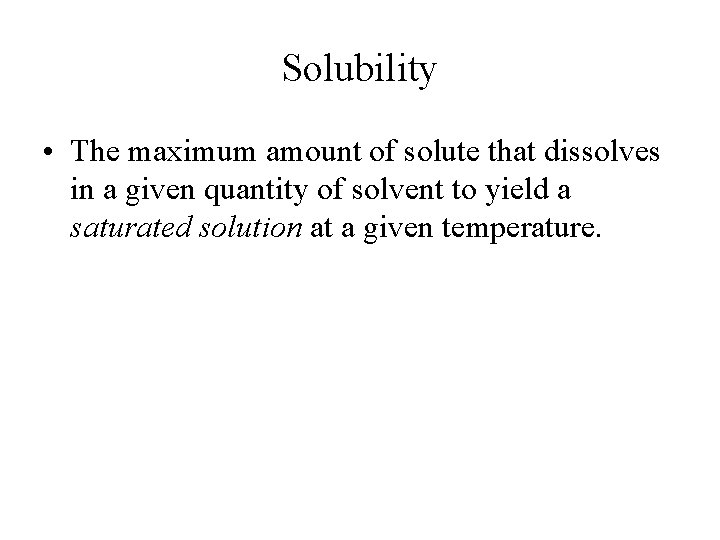Solubility • The maximum amount of solute that dissolves in a given quantity of solvent to yield a saturated solution at a given temperature.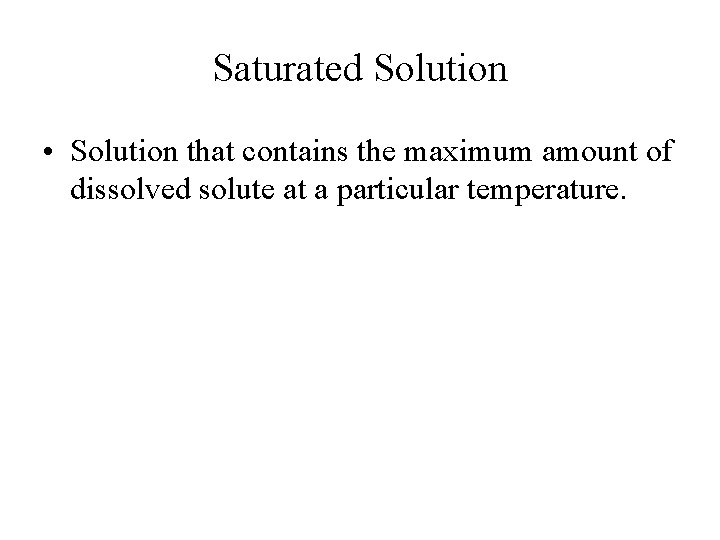Saturated Solution • Solution that contains the maximum amount of dissolved solute at a particular temperature.Supersaturated Solution • One that contains more dissolved solute than the maximum amount it normally has in the saturated solution.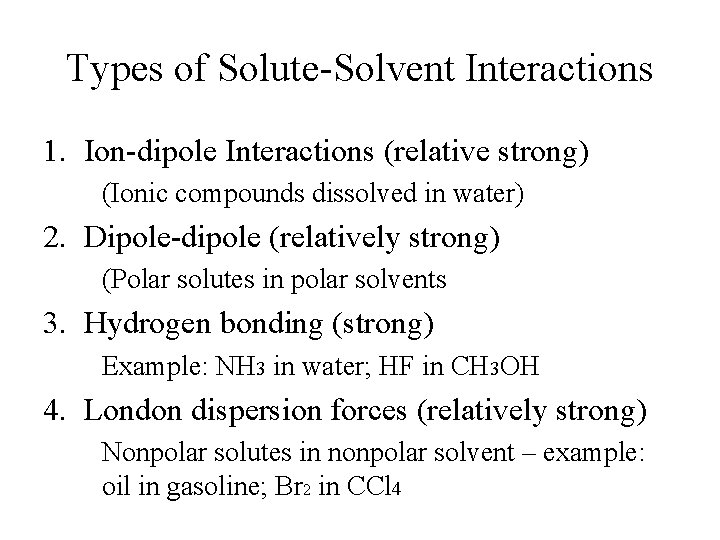Types of Solute-Solvent Interactions 1. Ion-dipole Interactions (relative strong) (Ionic compounds dissolved in water) 2. Dipole-dipole (relatively strong) (Polar solutes in polar solvents 3. Hydrogen bonding (strong) Example: NH 3 in water; HF in CH 3 OH 4. London dispersion forces (relatively strong) Nonpolar solutes in nonpolar solvent – example: oil in gasoline; Br 2 in CCl 4Other Types of Solute-Solvent Interactions • Ion-induced dipole (very weak) Ionic compounds in nonpolar solvent – example: Na. Cl in gasoline • Dipole-induced dipole (very weak) Nonpolar solute in polar solvent – example: iodine in water or alcohol Polar solute in nonpolar solvent – example: sugar in gasoline or hexane.Steps in the Formation of SolutionsFormation of a Liquid Solution Steps involved: 1. Separation of solute particles - an endothermic process (DH 1 > 0) 2. Separation of solvent molecules - an endothermic process (DH 2 > 0) 3. Mixing of solute and solvent to form the solution - an exothermic process (DH 3 < 0).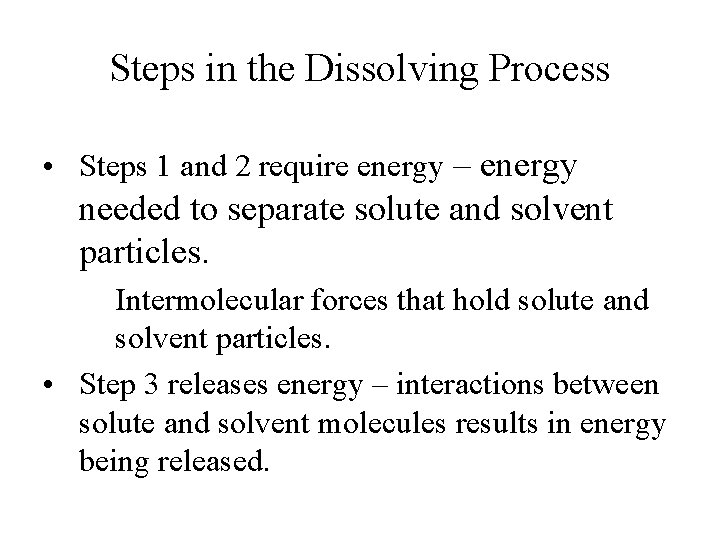Steps in the Dissolving Process • Steps 1 and 2 require energy – energy needed to separate solute and solvent particles. Intermolecular forces that hold solute and solvent particles. • Step 3 releases energy – interactions between solute and solvent molecules results in energy being released.Enthalpy of Solution • Enthalpy change associated with the formation of a solution is the sum of ΔH of each steps: ΔHsoln = ΔH 1 + ΔH 2 + ΔH 3 = ΔHsolute + ΔHsolvent + ΔHmixture • ΔHsoln may have a positive sign or a negative sign depends on magnitudes of individual ΔH.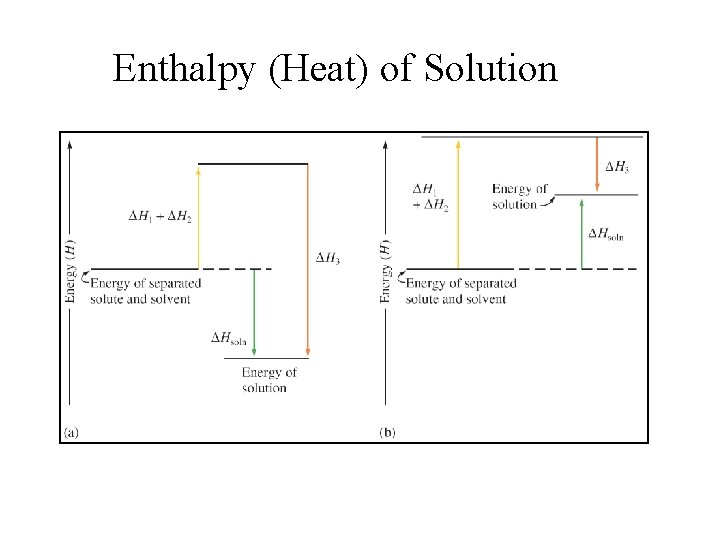Enthalpy (Heat) of Solution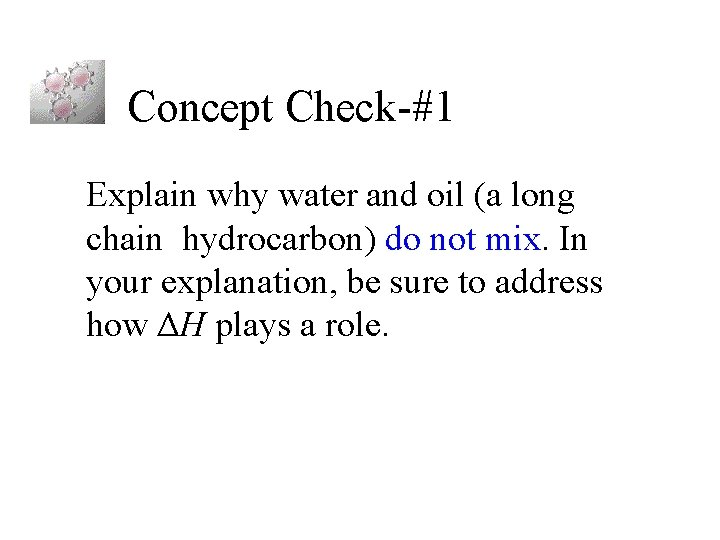Concept Check-#1 Explain why water and oil (a long chain hydrocarbon) do not mix. In your explanation, be sure to address how ΔH plays a role.Enthalpy of Hydration DHhydration = DHsolvent + DHmixing DHsolution = DHsolute + DHhydration: Mn+(g) + H 2 O Mn+(aq) Xn-(g) + H 2 O Xn-(aq) DHsolution = DHlattice + S(DHhydration )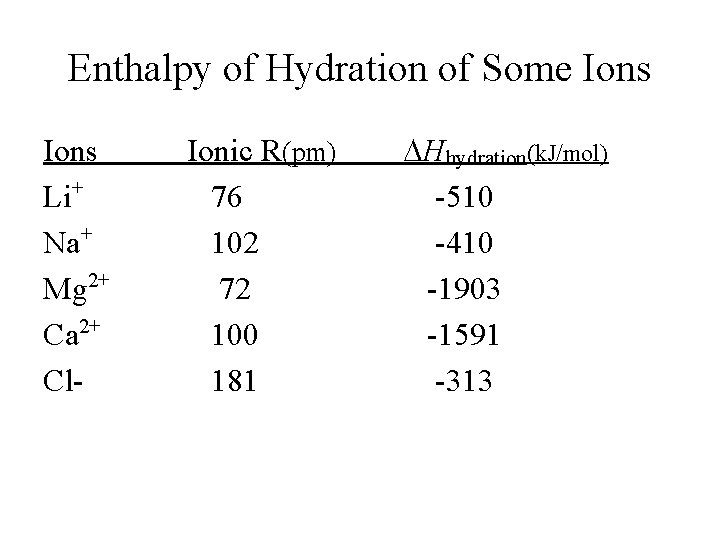Enthalpy of Hydration of Some Ions Li+ Na+ Mg 2+ Ca 2+ Cl- Ionic R(pm) 76 102 72 100 181 DHhydration(k. J/mol) -510 -410 -1903 -1591 -313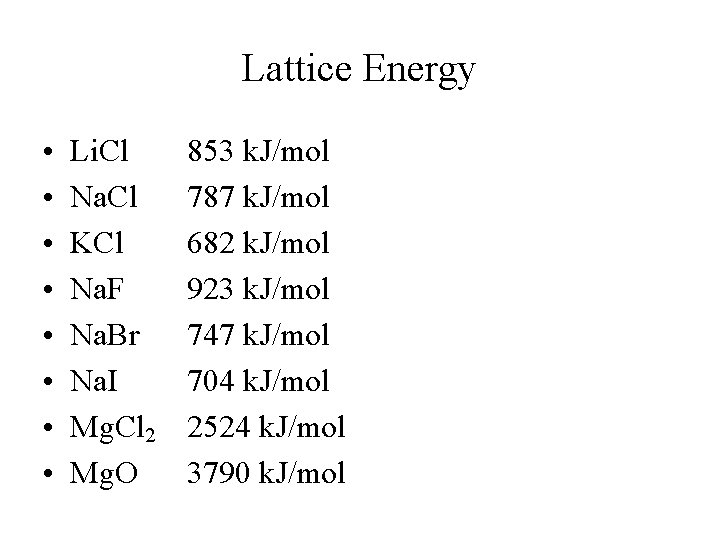Lattice Energy • • Li. Cl Na. Cl KCl Na. F Na. Br Na. I Mg. Cl 2 Mg. O 853 k. J/mol 787 k. J/mol 682 k. J/mol 923 k. J/mol 747 k. J/mol 704 k. J/mol 2524 k. J/mol 3790 k. J/mol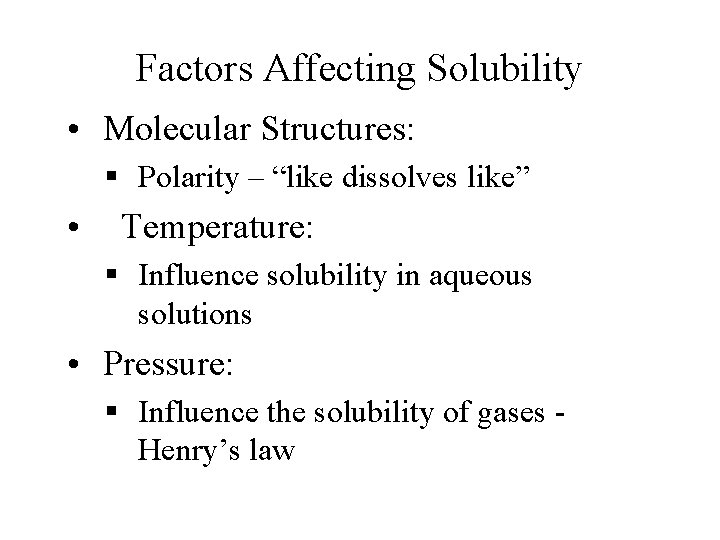Factors Affecting Solubility • Molecular Structures: § Polarity – “like dissolves like” • Temperature: § Influence solubility in aqueous solutions • Pressure: § Influence the solubility of gases Henry’s lawTemperature Effects • Solubility of most solids in water increases with temperature; very few exception. • Solubility of a gas in solvent typically decreases with increasing temperature. • Predicting the temperature dependence of solubility is very difficult.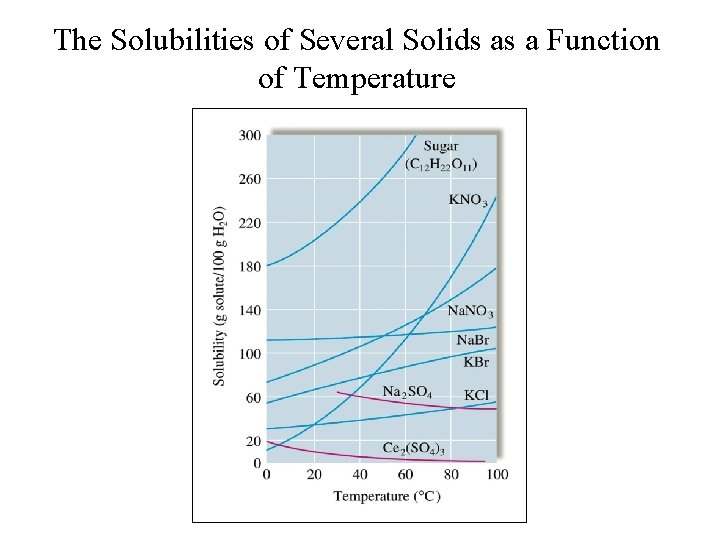The Solubilities of Several Solids as a Function of Temperature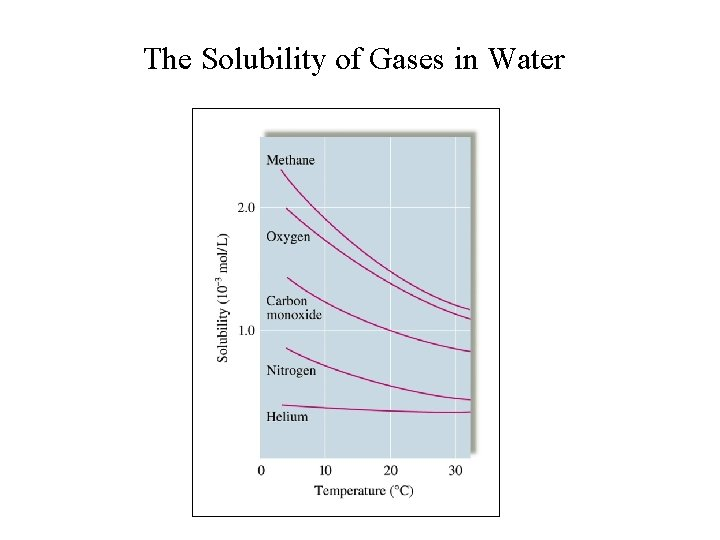The Solubility of Gases in WaterEffect of Pressure on Solubility • Henry’s law: c = k. P c = concentration of dissolved gas k = constant P = partial pressure of gas solute above the solution • Amount of gas dissolved in a solution is directly proportional to the partial pressure of gas above the solution.Effect of Pressure of Solubility of GasIdeal Solution: One that obeys Raoult’s Law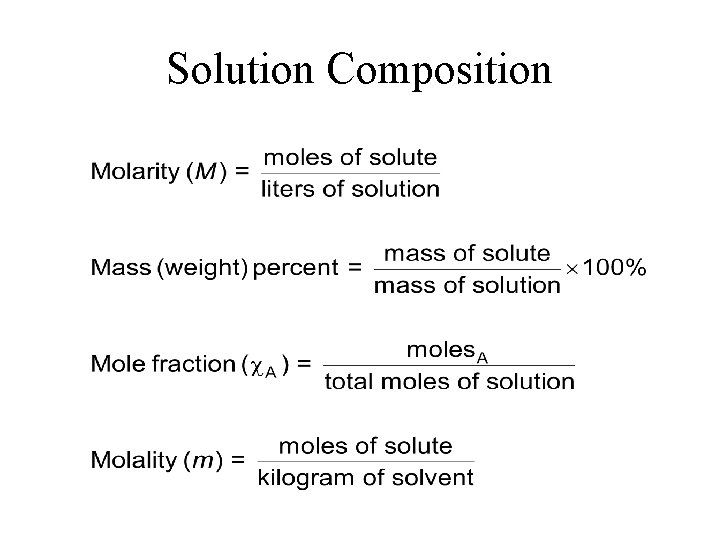Solution Composition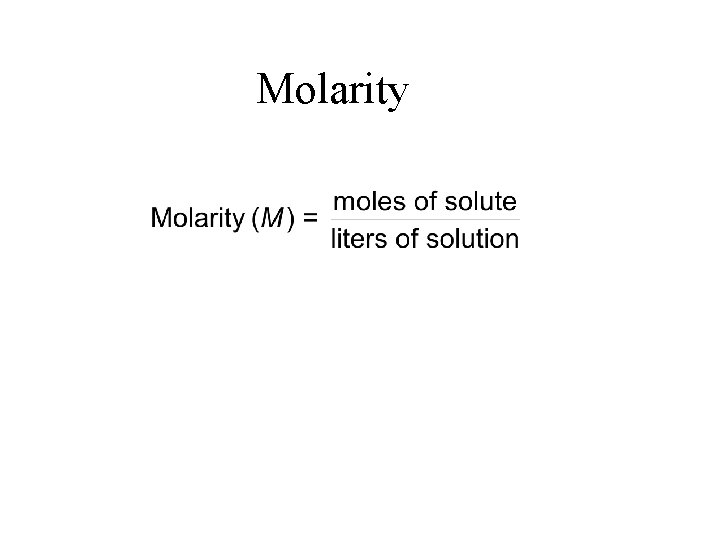Molarity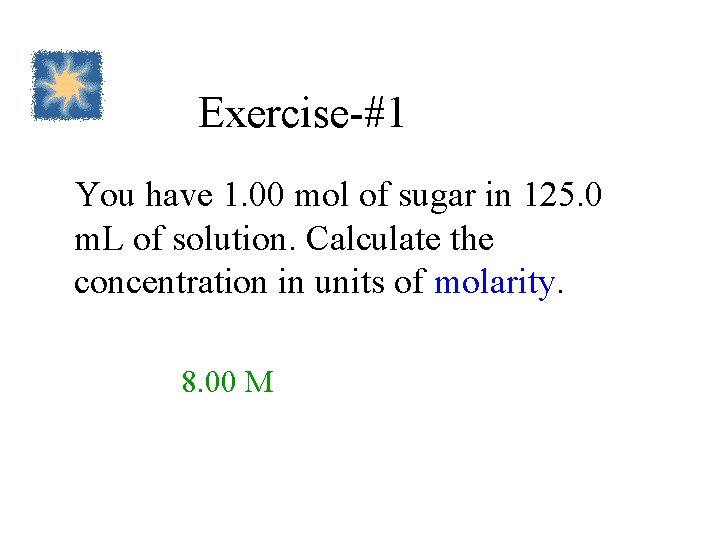Exercise-#1 You have 1. 00 mol of sugar in 125. 0 m. L of solution. Calculate the concentration in units of molarity. 8. 00 M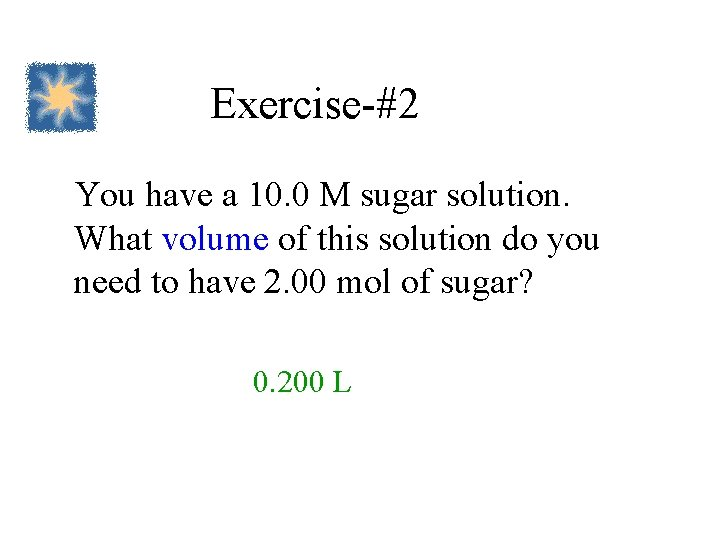Exercise-#2 You have a 10. 0 M sugar solution. What volume of this solution do you need to have 2. 00 mol of sugar? 0. 200 L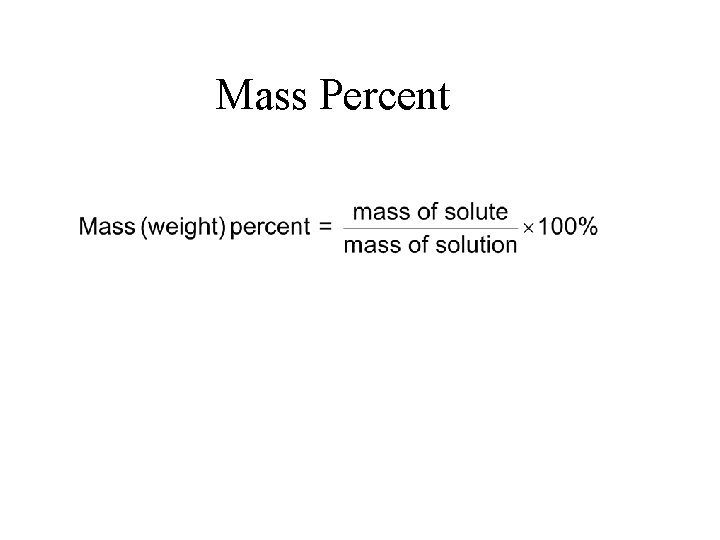Mass PercentExercise-#3 What is the percent-by-mass concentration of glucose in a solution made my dissolving 5. 5 g of glucose in 78. 2 g of water? 6. 6%Mole FractionExercise-#4 A solution of phosphoric acid was made by dissolving 8. 00 g of H 3 PO 4 in 100. 0 m. L of water. Calculate the mole fraction of H 3 PO 4. (Assume water has a density of 1. 00 g/m. L. ) 0. 0145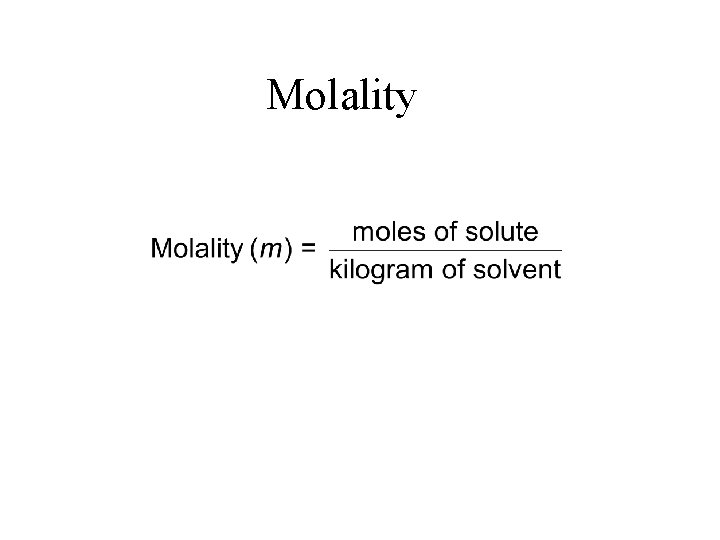Molality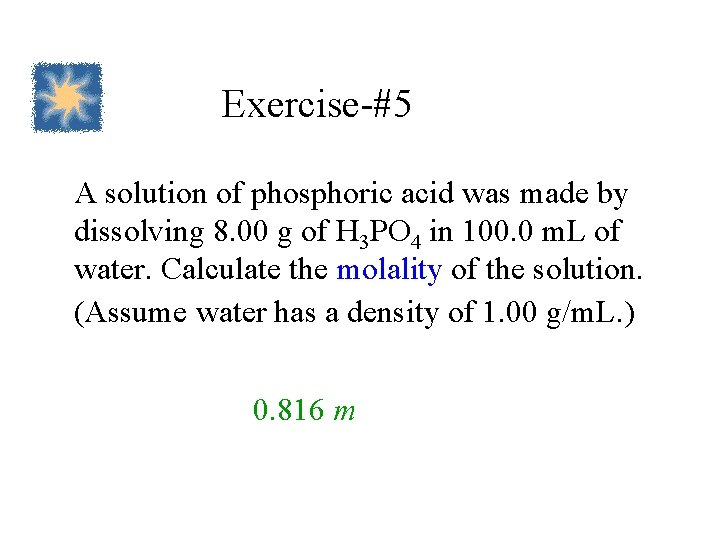Exercise-#5 A solution of phosphoric acid was made by dissolving 8. 00 g of H 3 PO 4 in 100. 0 m. L of water. Calculate the molality of the solution. (Assume water has a density of 1. 00 g/m. L. ) 0. 816 mSolutions containing Nonvolatile Solutes • Nonvolatile solute lowers the vapor pressure of solvent. • Raoult’s Law: Psoln = vapor pressure of solution solv = mole fraction of solvent = vapor pressure of pure solvent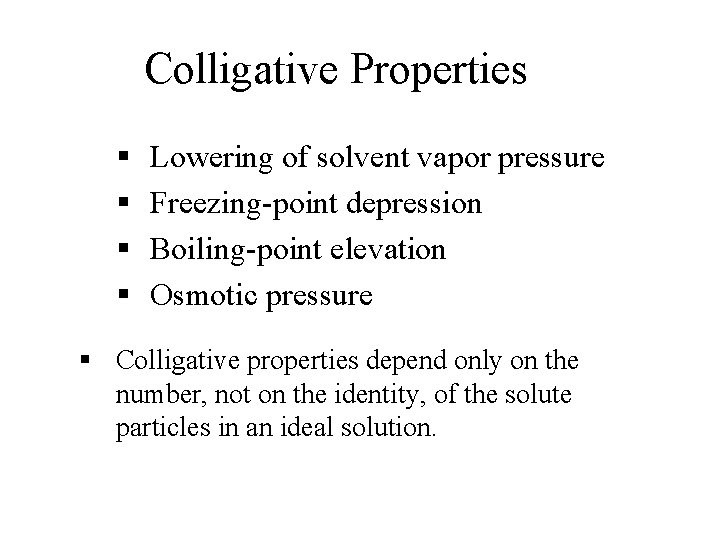Colligative Properties § § Lowering of solvent vapor pressure Freezing-point depression Boiling-point elevation Osmotic pressure § Colligative properties depend only on the number, not on the identity, of the solute particles in an ideal solution.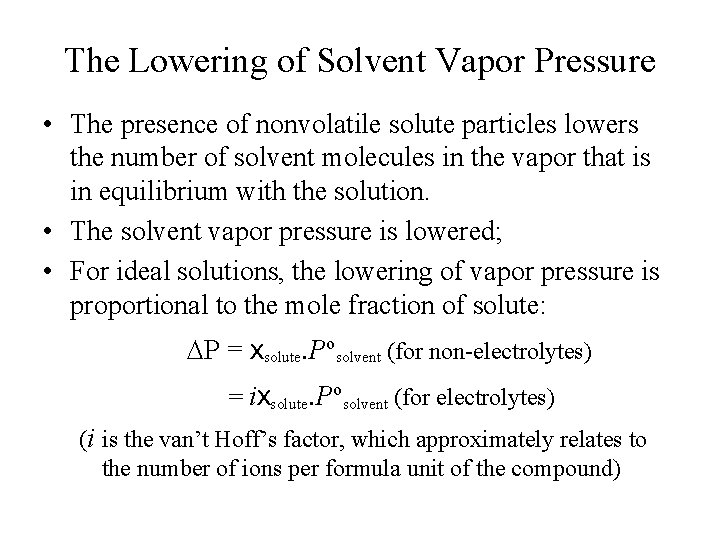The Lowering of Solvent Vapor Pressure • The presence of nonvolatile solute particles lowers the number of solvent molecules in the vapor that is in equilibrium with the solution. • The solvent vapor pressure is lowered; • For ideal solutions, the lowering of vapor pressure is proportional to the mole fraction of solute: DP = Xsolute. Posolvent (for non-electrolytes) = i. Xsolute. Posolvent (for electrolytes) (i is the van’t Hoff’s factor, which approximately relates to the number of ions per formula unit of the compound)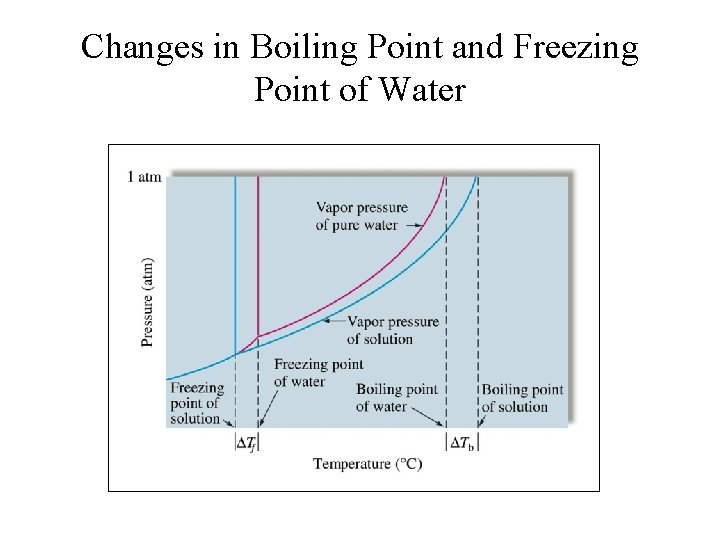Changes in Boiling Point and Freezing Point of Water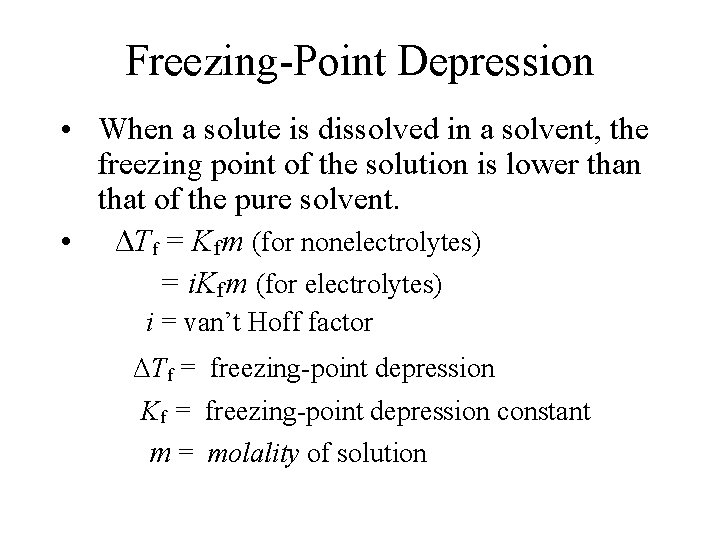Freezing-Point Depression • When a solute is dissolved in a solvent, the freezing point of the solution is lower than that of the pure solvent. • ΔTf = Kfm (for nonelectrolytes) = i. Kfm (for electrolytes) i = van’t Hoff factor ΔTf = freezing-point depression Kf = freezing-point depression constant m = molality of solution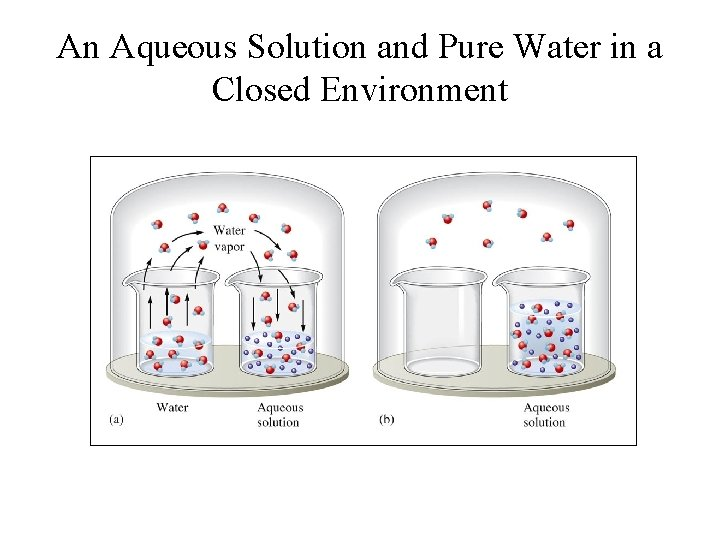An Aqueous Solution and Pure Water in a Closed EnvironmentLiquid-Vapor Equilibrium in Pure Solvent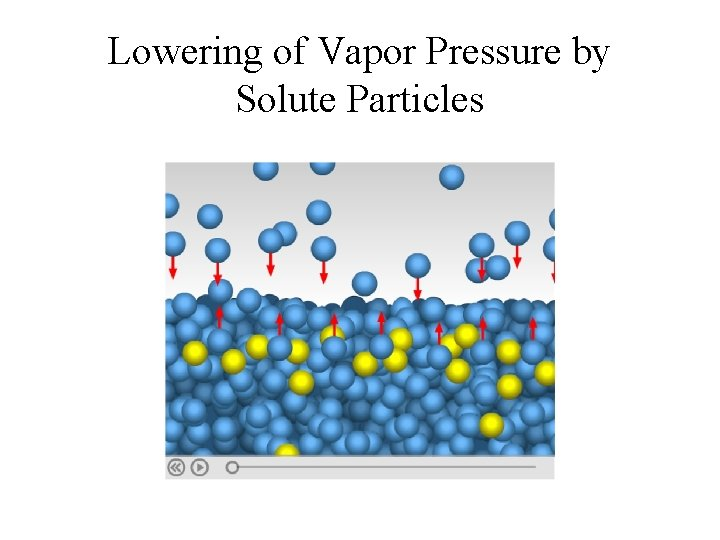Lowering of Vapor Pressure by Solute ParticlesSolute Particles Lower Solvent Vapor Pressure and Its Freezing PointBoiling-Point Elevation • Nonvolatile solute elevates the boiling point of the solvent. • ΔTb = Kbm (for nonelectrolytes) = i. Kbm (for electrolytes) i = van’t Hoff factor ΔTb = boiling-point elevation Kb = boiling-point elevation constant m = molality of solution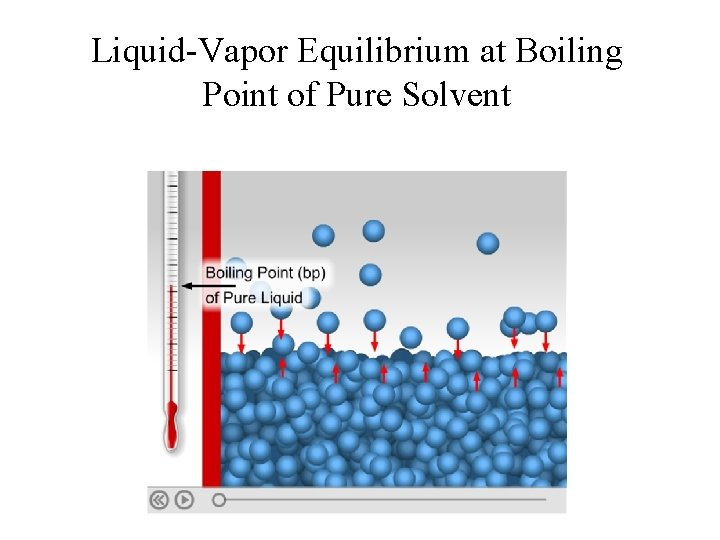Liquid-Vapor Equilibrium at Boiling Point of Pure Solvent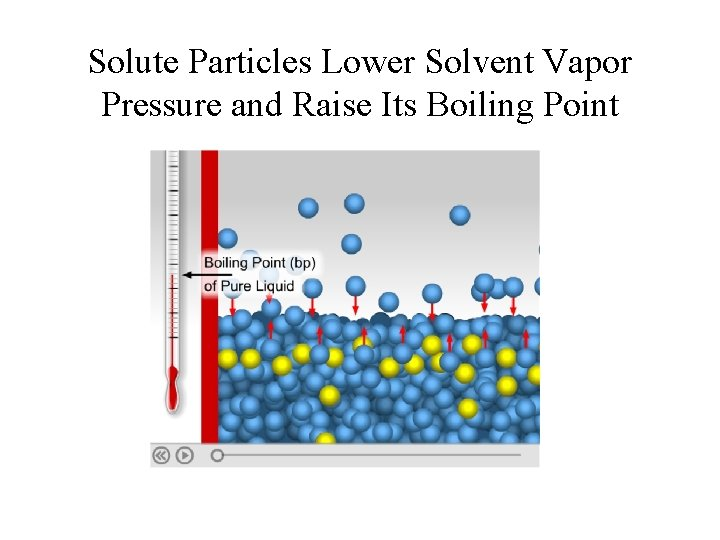Solute Particles Lower Solvent Vapor Pressure and Raise Its Boiling Point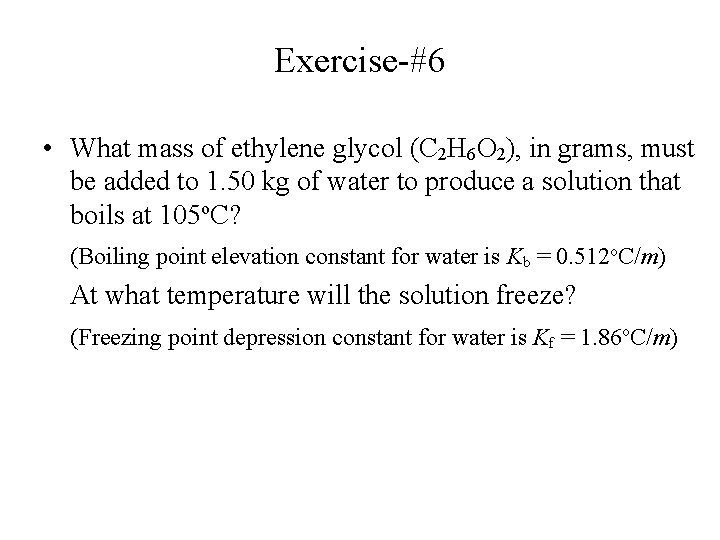Exercise-#6 • What mass of ethylene glycol (C 2 H 6 O 2), in grams, must be added to 1. 50 kg of water to produce a solution that boils at 105 o. C? (Boiling point elevation constant for water is Kb = 0. 512 o. C/m) At what temperature will the solution freeze? (Freezing point depression constant for water is Kf = 1. 86 o. C/m)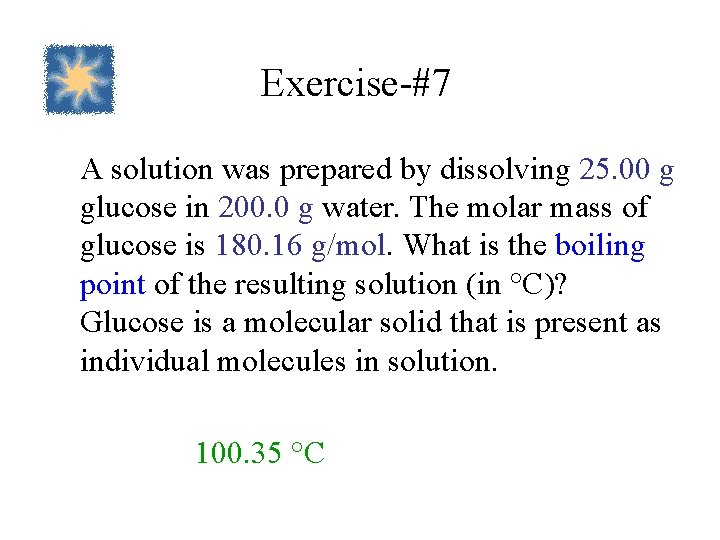Exercise-#7 A solution was prepared by dissolving 25. 00 g glucose in 200. 0 g water. The molar mass of glucose is 180. 16 g/mol. What is the boiling point of the resulting solution (in °C)? Glucose is a molecular solid that is present as individual molecules in solution. 100. 35 °CExercise-#8 Suppose you dissolve a 20. 0 -g mixture containing sucrose (C 12 H 22 O 11) and Na. Cl in 1. 0 kg of water. The solution is found to freeze at -0. 426°C. (a) Assuming ideal behavior, calculate the mass percent of sucrose and Na. Cl, respectively, in the mixture. (b) Calculate the mole fraction of sucrose in the mixture. (a) Mass %: sucrose = 72. 8%; Na. Cl = 27. 2%; (b) Mole fraction of sucrose = 0. 313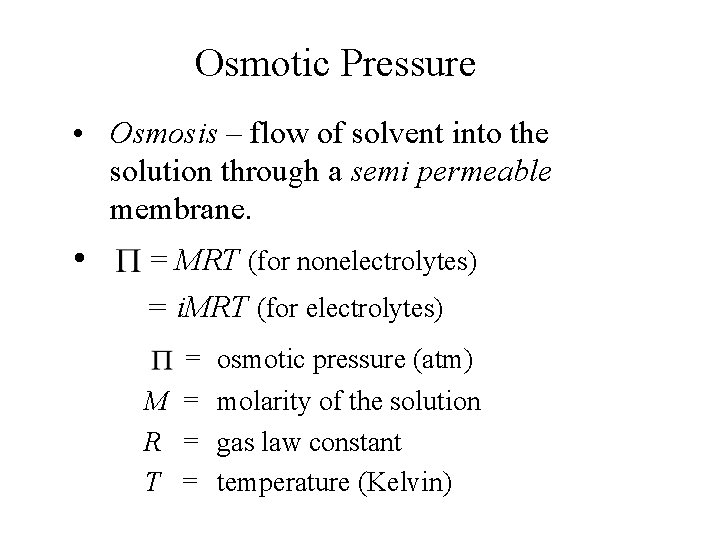Osmotic Pressure • Osmosis – flow of solvent into the solution through a semi permeable membrane. • = MRT (for nonelectrolytes) = i. MRT (for electrolytes) = osmotic pressure (atm) M = molarity of the solution R = gas law constant T = temperature (Kelvin)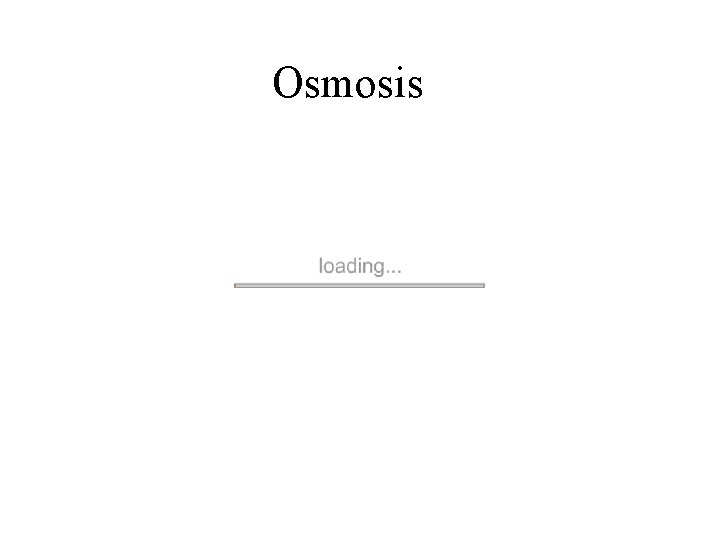Osmosis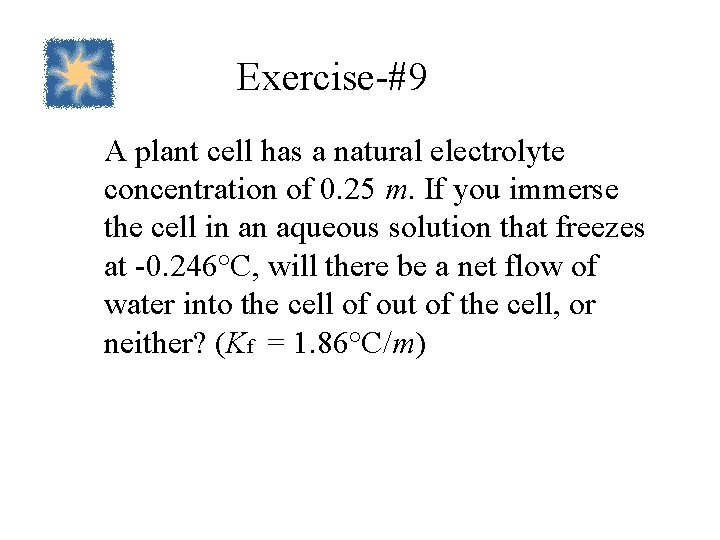Exercise-#9 A plant cell has a natural electrolyte concentration of 0. 25 m. If you immerse the cell in an aqueous solution that freezes at -0. 246°C, will there be a net flow of water into the cell of out of the cell, or neither? (Kf = 1. 86°C/m)Exercise-#10 When a 33. 4 -mg sample of a compound is dissolved in 20. 0 m. L of water at 25°C, the solution has an osmotic pressure of 58. 0 torr. Calculate the molar mass of this compound. (Assume the compound is nonelectrolyte. ) 535 g/mol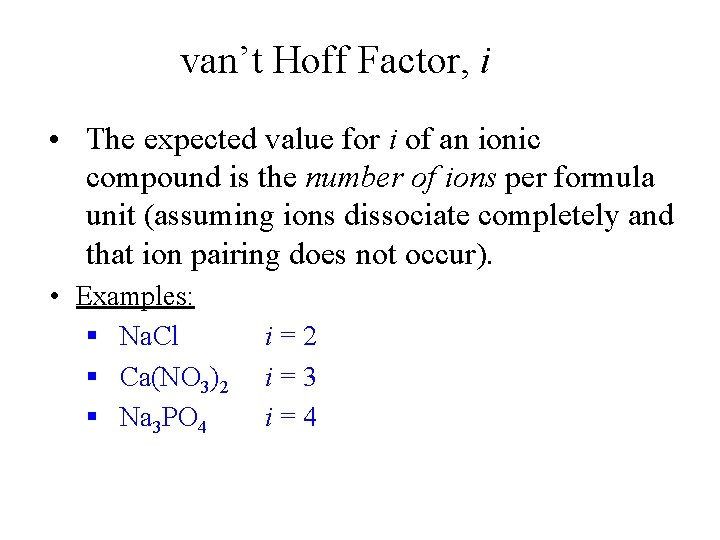van’t Hoff Factor, i • The expected value for i of an ionic compound is the number of ions per formula unit (assuming ions dissociate completely and that ion pairing does not occur). • Examples: § Na. Cl § Ca(NO 3)2 § Na 3 PO 4 i=2 i=3 i=4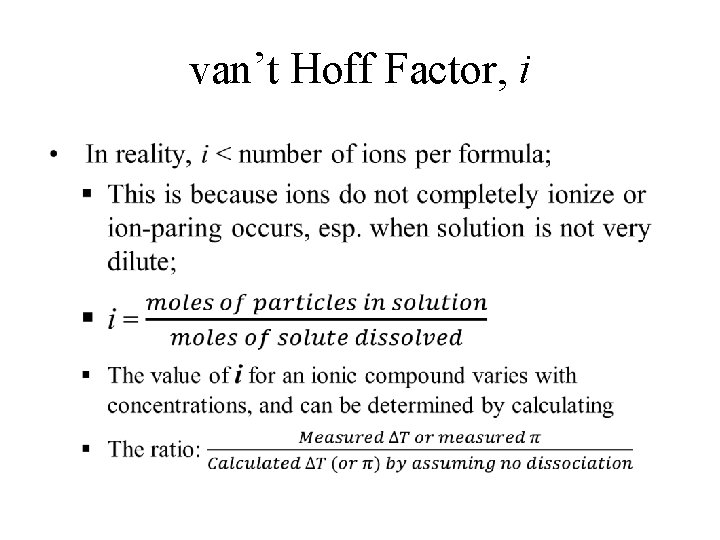van’t Hoff Factor, i •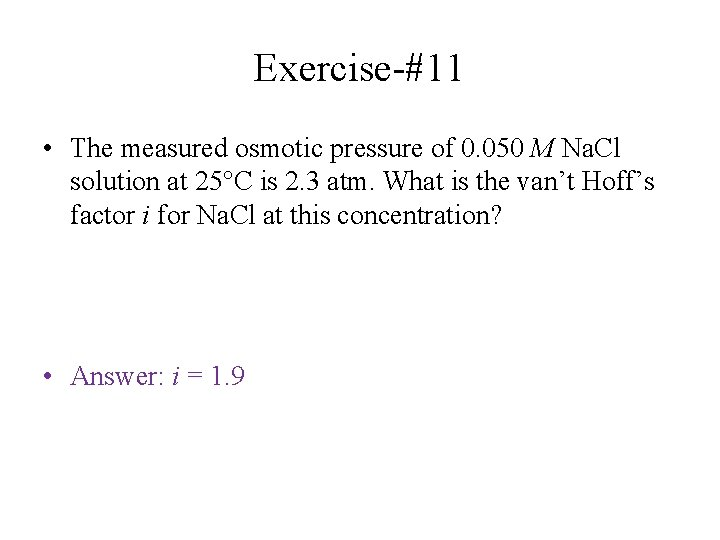Exercise-#11 • The measured osmotic pressure of 0. 050 M Na. Cl solution at 25°C is 2. 3 atm. What is the van’t Hoff’s factor i for Na. Cl at this concentration? • Answer: i = 1. 9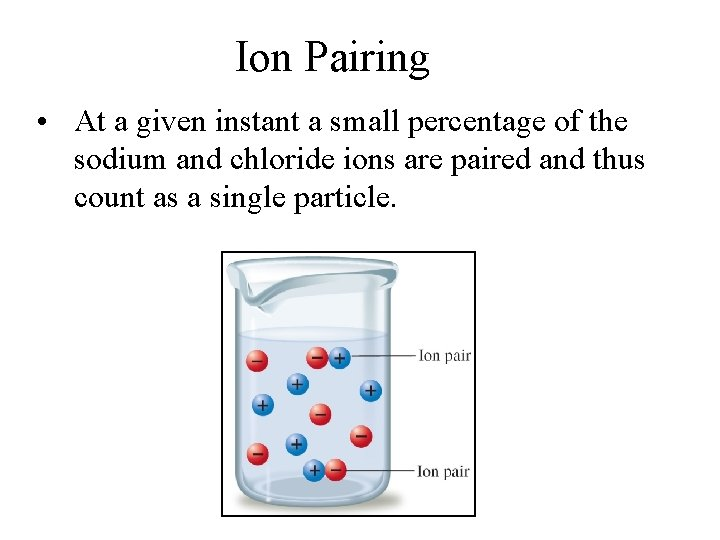Ion Pairing • At a given instant a small percentage of the sodium and chloride ions are paired and thus count as a single particle.Ion Pairing • Ion-pairing occurs to some extent in all electrolyte solutions. • They are more significant in concentrated solutions. • Ion-pairings are also significant in solutions containing highly charged ions. • Ion-parings are less significant in very dilute solutions.Solutions Composed of Two Liquids • Two Liquids form Ideal Solution if: – they are structurally very similar, and – interactions between non-identical molecules were relatively similar to identical molecules. • The vapor of each liquid obeys Raoult’s Law: PA = XAPo. A; P B = X B P o. B PT = PA + PB = XAPo. A + XBPo. B (X : mole fraction; Po : vapor pressure of pure liquid)A Summary of the Behavior of Various Types of Solutions Composed of Two Volatile Liquids Interactive Forces Between Solute (A) and Solvent (B) Particles Hsoln T for Solution Formation Deviation from Raoult’s Law Example Benzenetoluene A A, B B A B Zero None (ideal solution) A A, B B < A B Negative (exothermic) Positive Negative Acetonewater A A, B B > A B Positive (endothermic) Negative Positive EthanolhexaneVapor Pressure for a Solution of Two Volatile Liquids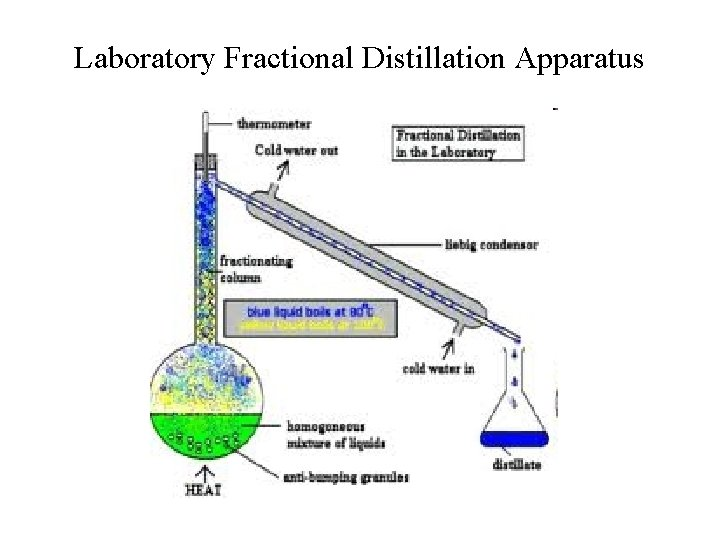Laboratory Fractional Distillation ApparatusFractional Distillation Towers in Oil Refinaries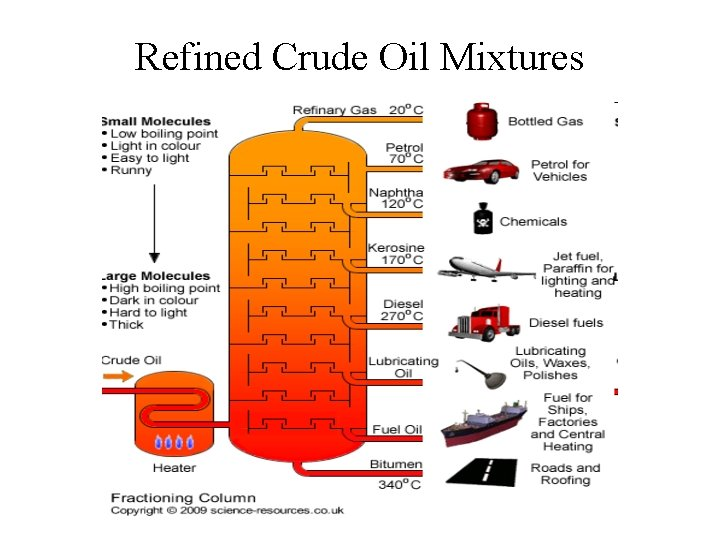Refined Crude Oil Mixtures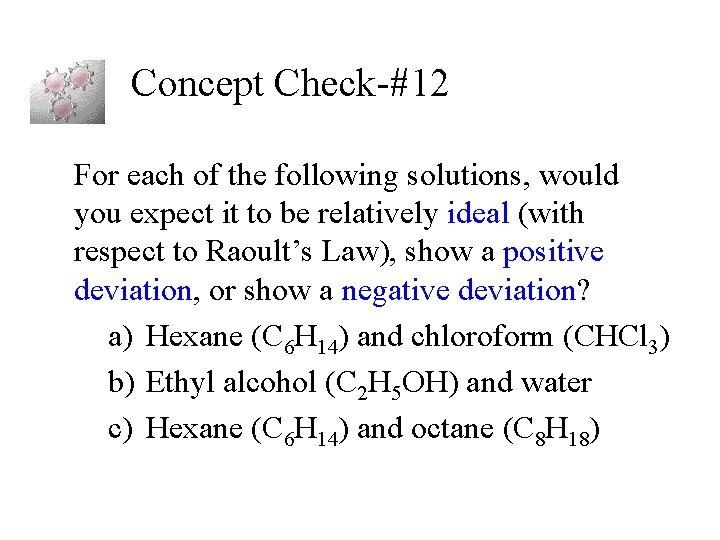Concept Check-#12 For each of the following solutions, would you expect it to be relatively ideal (with respect to Raoult’s Law), show a positive deviation, or show a negative deviation? a) Hexane (C 6 H 14) and chloroform (CHCl 3) b) Ethyl alcohol (C 2 H 5 OH) and water c) Hexane (C 6 H 14) and octane (C 8 H 18)Exercise-#13 • A solution of benzene (C 6 H 6) and toluene (C 7 H 8) contains 50. 0% benzene by mass. The vapor pressures of pure benzene and pure toluene at 25 o. C are 95. 1 torr and 28. 4 torr, respectively. Assuming ideal behavior, calculate the following: (a) The mole fractions of benzene and toluene; (b) The total vapor pressure above the solution; (c) The mole percent of each component in the vapor.Exercise-#14 • A solution made up of 24. 3 g acetone (CH 3 COCH 3) and 39. 5 g of carbon disulfide (CS 2) has a vapor pressure of 645 torr at 35 o. C. The vapor pressures of pure acetone and pure carbon disulfide at 35 o. C are 332 torr and 515 torr, respectively. (a) Is the solution ideal or non-ideal? (b) If non-ideal, does it deviate positively or negatively from Raoult’s law? (c) What can you say about the relative strength of carbon disulfide-acetone interactions compared to the acetone and carbon disulfide-carbon disulfide interactions?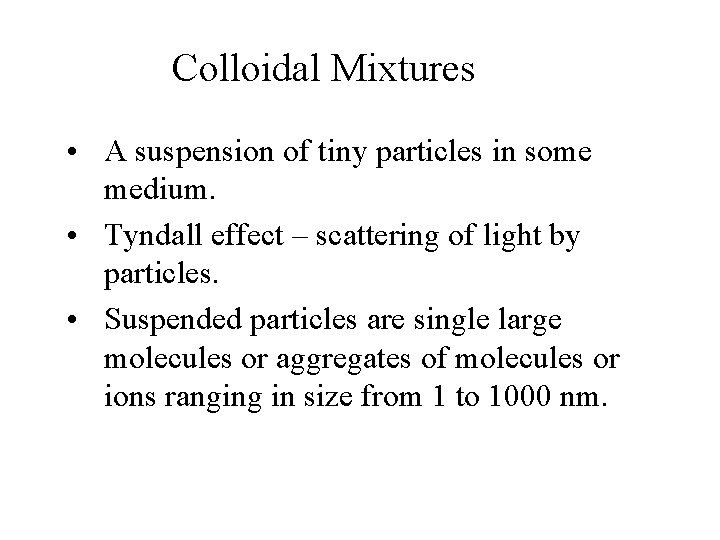Colloidal Mixtures • A suspension of tiny particles in some medium. • Tyndall effect – scattering of light by particles. • Suspended particles are single large molecules or aggregates of molecules or ions ranging in size from 1 to 1000 nm.Scattering of Light by Colloid Particles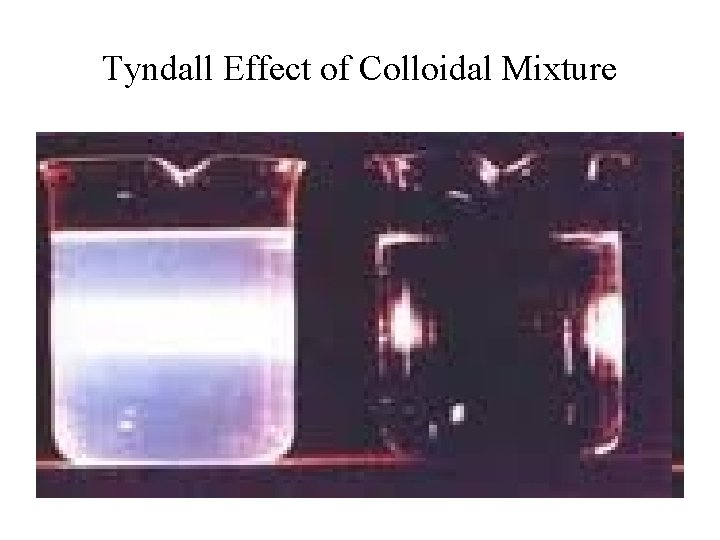Tyndall Effect of Colloidal Mixture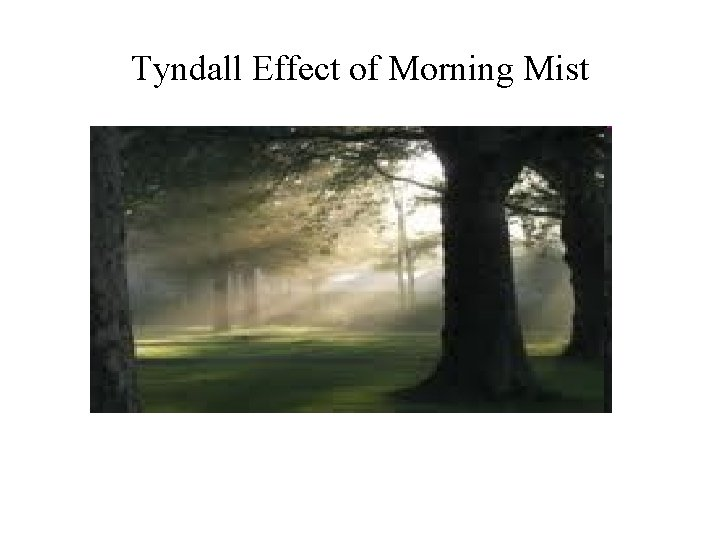Tyndall Effect of Morning Mist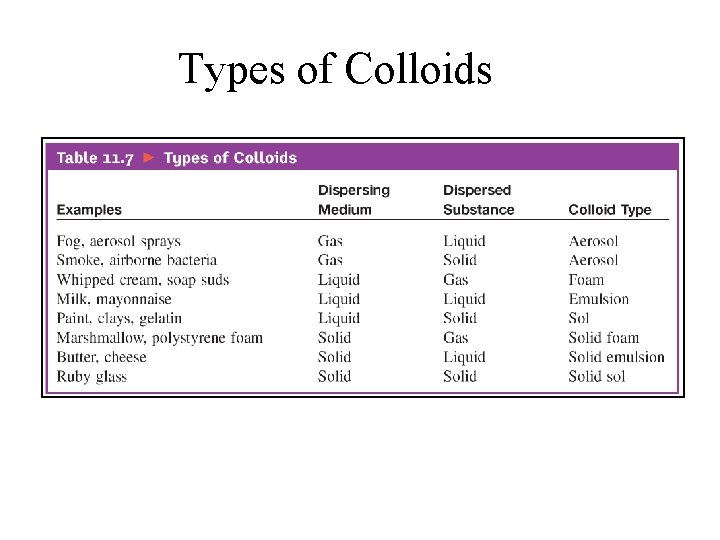Types of ColloidsMicelle – A Colloidal Suspension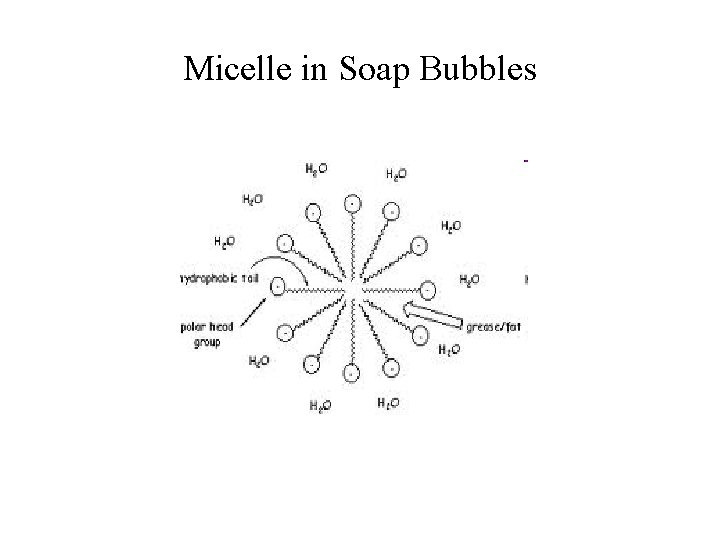Micelle in Soap Bubbles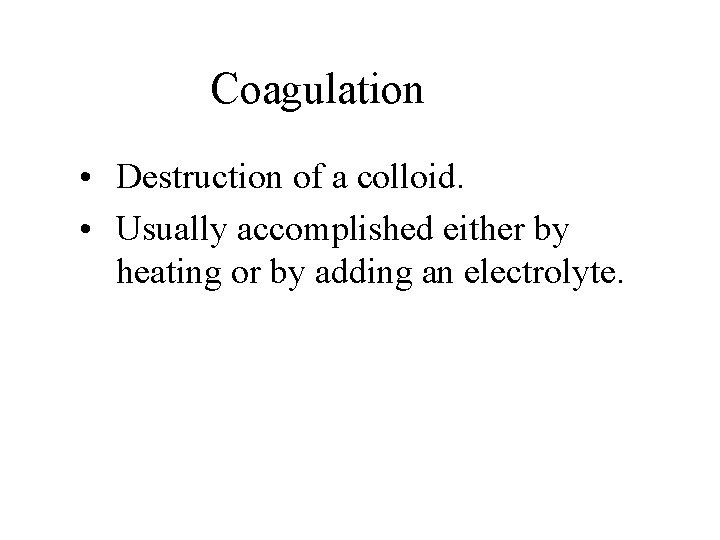Coagulation • Destruction of a colloid. • Usually accomplished either by heating or by adding an electrolyte.